# Dart Loops Tutorial with Examples

#### View more Tutorials: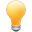Follow us on our fanpages to receive notifications every time there are new articles.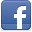Facebook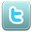Twitter

## 1- What is loop?

In programming languages, loops allow a block of code to be executed repeatedly.
The Dart language provides the following types of loops:
• for
• for..in
• while
• do..while
The break and continue statements can appear inside the loop:
break
When the break statement is encountered, the program will terminate the loop immediately.
continue
When the continue statement is encountered, the program will skip the lines below the continue and execute the next iteration (If the loop condition is still true).

## 2- for loop

Syntax of for loop:
``````
for (initialValues; condition; updateValues) {
// Statements to be executed repeatedly
}
``````
• InitialValues: Initialize values for related variables in the loop.
• condition: Condition to execute the block.
• updateValues: Update new values for variables.
Example:
loop_for_ex1.dart
``````
void main() {
print('For loop example');

for (var i = 0; i < 10; i = i + 3) {
print('i= \$i');
}
}
``````
Output:
``````
For loop example
i= 0
i= 3
i= 6
i= 9
``````
Example: Two variables participate in the condition of a for loop:
loop_for_ex2.dart
``````
void main() {
print('For loop example');

for (var i = 0, j = 0; i + j < 10; i = i + 1, j = j + 2) {
print('i = \$i, j = \$j');
}
}
``````
Output:
``````
For loop example
i = 0, j = 0
i = 1, j = 2
i = 2, j = 4
i = 3, j = 6
``````

## 3- for..in loop

The for..in loop is used to iterate over the elements of an Iterable.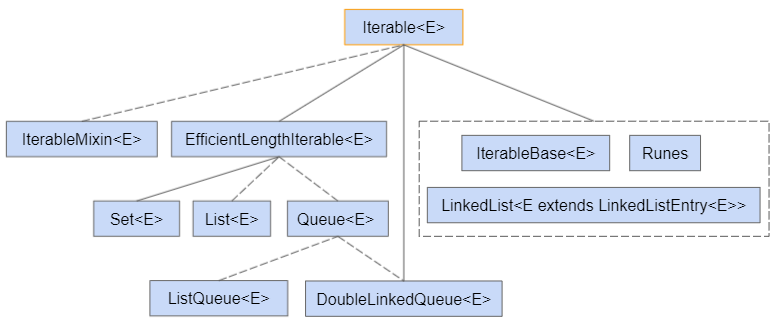In the Dart programming language, List is a descendant of Iterable, which has the same feature as an array of other programming languages.
Example: Use for..in loop to iterate over the elements of a List:
loop_for_in_list_ex1.dart
``````
void main() {
// Create a List:
var names = ['Tom', 'Jerry', 'Donald'];

for (var name in names) {
print('name: \$name');
}
}
``````
Output:
``````
name: Tom
name: Jerry
name: Donald
``````
Example: Use for..in loop to iterate over the elements of a Set object.
loop_for_in_set_ex1.dart
``````
void main() {
// Create a Map object:
// (key, value) <--> (Emloyee Number, Salary).
var salaryMap = {'E01': 1200,
'E02': 1500,
'E03': 900};
// The Set object.
var keySet = salaryMap.keys;

for (var key in keySet) {
print('Employee Number: \$key, salary: \${salaryMap[key]}');
}
}
``````
Output:
``````
Employee Number: E01, salary: 1200
Employee Number: E02, salary: 1500
Employee Number: E03, salary: 900
``````

## 4- while loop

while loop syntax:
``````
while (condition)  {
// Do something here..
}
``````
Example:
loop_while_ex1.dart
``````
void main() {
print('While loop example');
var x = 2;
while (x < 10) {
print('Value of x = \$x');
x = x + 3;
}
print('Done!');
}
``````
Output:
``````
While loop example
Value of x = 2
Value of x = 5
Value of x = 8
Done!
``````

## 5- do..while loop

The do..while loop is used to execute a program segment multiple times. The characteristics of do..while is that a block of code is always executed at least once. After each iteration, the program checks the condition again, if the condition is still true, the next iteration will be executed.
The do..while loop syntax:
``````
do {
// Do something
}
while(condition);
``````
Example:
loop_do_while_ex1.dart
``````
void main() {
var value = 3;
do {
print('Value = \$value');
value = value + 3;
} while (value < 10);
}
``````
Output:
``````
Value = 3
Value = 6
Value = 9
``````

## 6- break statement in loop

break is a statement that can appear in the block of a loop. This is the statement to unconditionally terminate the loop.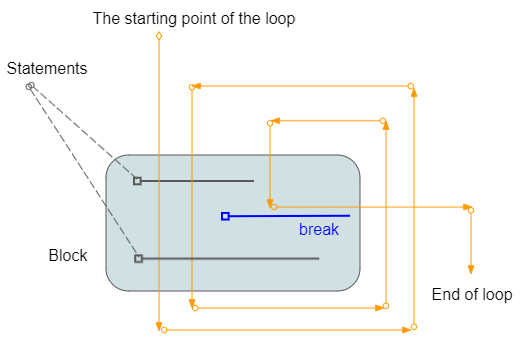Example:
loop_break_ex1.dart
``````
void main() {
print('Break example');
var x = 2;
while (x < 15) {
print('----------------------');
print('x = \$x');
// If y = 5 then exit the loop.
if (x == 5) {
break;
}
// Increase value of x by 1
x = x + 1;
print('x after + 1 = \$x');
}
print('Done!');
}
``````
Output:
``````
Break example
----------------------
x = 2
x after + 1 = 3
----------------------
x = 3
x after + 1 = 4
----------------------
x = 4
x after + 1 = 5
----------------------
x = 5
Done!
``````

## 7- continue statement in loop

continue is a statement that can appear in a loop. When the continue statement is encountered, the program will skip the lines below the continue and start a new iteration (If the condition is still true).Example:
loop_continue_ex1.dart
``````
void main() {
print('Continue example');
var x = 2;
while (x < 7) {
print('----------------------');
print('x = \$x');

if (x % 2 == 0) {
x = x + 1;
continue;
} else {
x = x + 1;
print('x after + 1 = \$x');
}
}
print('Done!');
}
``````
Output:
``````
Continue example
----------------------
x = 2
----------------------
x = 3
x after + 1 = 4
----------------------
x = 4
----------------------
x = 5
x after + 1 = 6
----------------------
x = 6
Done!
``````

## 8- Labeled Loops

Dart allows you to put a label for a loop. This is a way to name a loop and comes in handy when you use multiple nested loops in a program.
• You can use the "break labelX" statement to break the loop labeled labelX.
• You can use the "continue labelX" statement to continue the loop labeled labelX.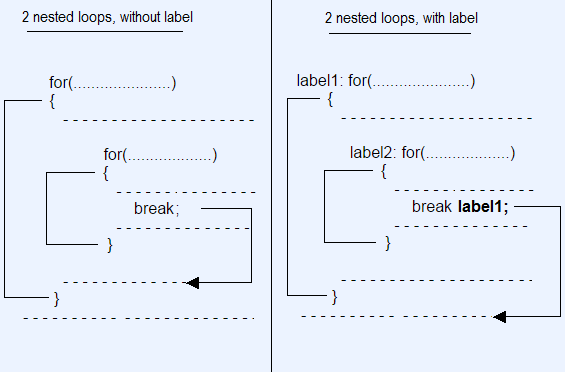Syntax for declaring a loop with labels.
``````
// for loop with Label.
label1: for( ... )  {

}
// while loop with Label.
label2: while ( ... )  {

}
// do-while loop with Label.
label3: do  {

} while ( ... );
``````
Example: Use labeled loops and break statement.
loop_break_labelled_ex1.dart
``````
void main() {
print('Labelled Loop Break example');
var i = 0;
label1:
while (i < 5) {
print('----------------------');
print('i = \$i');
i++;
label2:
for (var j = 0; j < 3; j++) {
print('  --> \$j');
if (j > 0) {
// Exit the loop with label1.
break label1;
}
}
}
print('Done!');
}
``````
Output:
``````
Labelled Loop Break example
----------------------
i = 0
--> 0
--> 1
Done!
``````
Example: Use labeled loops and continue statement.
loop_continue_labelled_ex1.dart
``````
void main() {
var i = 0;
label1:
while (i < 5) {
print('outer i=  \$i');
i++;
label2:
for (var j = 0; j < 3; j++) {
if (j > 0) {
continue label2;
}
if (i > 1) {
continue label1;
}
print('inner i= \$i, j= \$j');
}
}
}
``````
Output:
``````
outer i=  0
inner i= 1, j= 0
outer i=  1
outer i=  2
outer i=  3
outer i=  4
``````

#### View more Tutorials:

Maybe you are interested

These are online courses outside the o7planning website that we introduced, which may include free or discounted courses.

•Google DART Programming for Android- BOOTCAMP
•The Complete 2020 Flutter Development Bootcamp with Dart
•Dart 2 Complete Bootcamp - Go Hero from Zero in Dart Flutter
•Understand Concepts of DART Programming quickly and easily!
•Learning Dart: Become more productive with Dart
•AngularDart - Build Dynamic Web Apps with Angular & Dart
•Dart Masterclass Programming Course: iOS/Android Bible
•Dart Programming from Beginner to Expert
•Flutter & Dart - The Complete Guide [2020 Edition]
•Beginning Web Components with Dart
•Learn Dart Programming for Flutter
•Master Flutter - Learn Dart & Flutter by Developing 20 Apps
•Dart 2 Programming Bootcamp For Complete Beginners
•Dart and Flutter: The Complete Developer's Guide
•Complete Dart programming
•Flutter & Dart: A Complete Showcase Mobile App™
•Google DART Programming HANDS-ON with PYTHON File Handling
•Introducing Dart
•Flutter & Dart - The Complete Flutter App Development Course
•Learn Dart 2 in 90 minutes
•Flutter & Dart Development For Building iOS and Android Apps
•AWS Mobile with Google Dart
•The Complete Flutter UI Masterclass | iOS & Android in Dart
•Dart & Flutter: The Complete Mobile Apps Development Course
•Dart and Flutter From Zero to Hero - Practical Dev Bootcamp